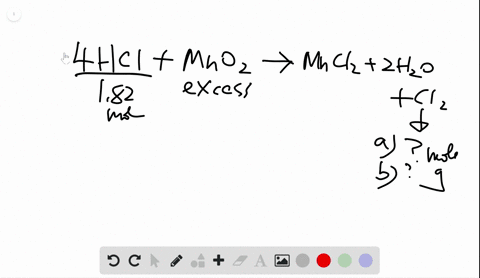🎉 The Study-to-Win Winning Ticket number has been announced! Go to your Tickets dashboard to see if you won! 🎉View Winning Ticket### Chlorine gas can be made in the laboratory by the…

02:10University of Maine
Problem 67

# Reactants D and E form product F. Draw a road map and write a Plan to find the mass (g) of F when 27 g of D reacts with 31 g of E.

## Discussion

You must be signed in to discuss.

## Video Transcript

in a chemical reaction. When you're given quantities of reactant, you confined the amount of product using strike you metric Lee equivalent ratios. It's helpful to draw a visual diagram or a road map to identify the different conversions needed along the way. Okay, in a problem where you're given quantities of both reactant. So, for example, in this reaction where D plus E reacts to form F, you're given 27 grams of D and 31 grams of E. One of these reactant is what's called the limiting re agent or the one that makes the amount of product. The other is in excess, which means that some will remain after reacting. So to figure out which one is the limiting re agent, we need to use both to calculate how much product would form. So we start off with their grams of each substance and change each one of those two moles, and we do this by dividing by the molar mass. Once we have our quantities in moles, we can find out how much product will form or how many moles of F. This information is found using the mole ratio from the balanced equation of these 21 will be smaller, so one will produce less product using the smaller amount. We can change it to grams and we do that by multiplying by the molar mass of a.